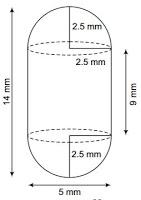Newbie

# A medicine capsule is in the shape of a cylinder with two hemispheres stuck to each of its ends. The length of the entire capsule is 14 mm and the diameter of the capsule is 5 mm. Find its surface area Q.6

• 0

Find is the solution for class 10 ncert chapter surface areas and volumes . Find the best solution of the exercise 13.1 question number 6 give me the easiest and simplest solution of this question .A medicine capsule is in the shape of a cylinder with two hemispheres stuck to each of its ends. The length of the entire capsule is 14 mm and the diameter of the capsule is 5 mm. Find its surface area

Share

1. Two hemisphere and one cylinder are shown in the figure given below.Here, the diameter of the capsule = 5 mm

∴ Radius = 5/2 = 2.5 mm

Now, the length of the capsule = 14 mm

So, the length of the cylinder = 14-(2.5+2.5) = 9 mm

∴ The surface area of a hemisphere = 2πr2 = 2×(22/7)×2.5×2.5

= 275/7 mm2

Now, the surface area of the cylinder = 2πrh

= 2×(22/7)×2.5×9

(22/7)×45 = 990/7 mm2

Thus, the required surface area of medicine capsule will be

= 2×surface area of hemisphere + surface area of the cylinder

= (2×275/7) × 990/7

(550/7) + (990/7) = 1540/7 = 220 mm2

• 0